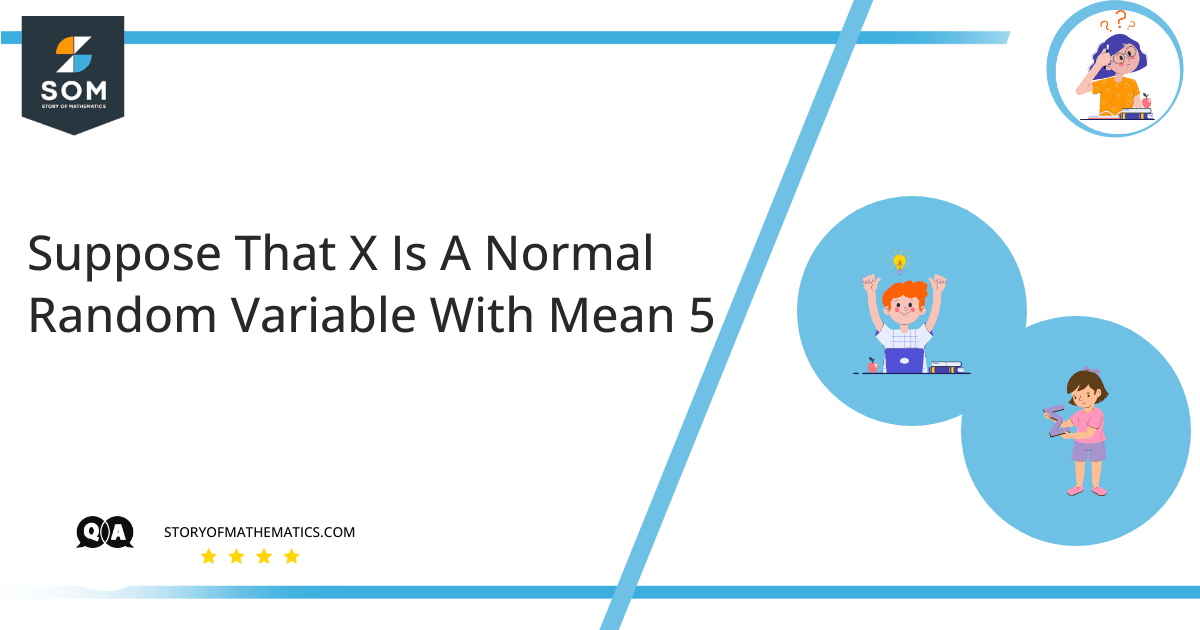# Suppose that X is a normal random variable with mean 5. If P(X>9)=0.2, approximately what is Var(X)?This question aims to find the probability of a normally distributed random variable $X$. A random variable is one whose value is determined by the results of a statistical experiment.

The normal distribution, also known as the Gaussian distribution or the z-distribution, has a mean of zero and a standard deviation of one. Data in a normal distribution is symmetrically distributed and has no skew. The data takes the shape of a bell when plotted on a graph, with most values grouping around a central region and scattering off as they move away from the center.

The two characteristics such as mean and standard deviation define the graph of the normal distribution. The mean/average is the maximum of the graph, whereas the standard deviation measures the amount of spread away from the mean.

Let $\mu$ and $\sigma$ be the mean and standard deviation of the random variable $X$. According to the question:

$\mu=5$, $P(X>9)=0.2$  and  we have to find  Var(X) $=\sigma^2$.

Since, $P(X>9)=0.2$

$\implies P(X<9)=1-0.2=0.8$

$\implies P\left(Z<\dfrac{x-\mu}{\sigma}\right)=0.8$

$\implies P\left(Z<\dfrac{9-5}{\sigma}\right)=0.8$

$\implies \phi\left(\dfrac{9-5}{\sigma}\right)=0.8$

So, by inverse use of the $z-$ table, when $\phi(z)=0.8$ then $z\approx 0.84$. And hence:

$\dfrac{9-5}{\sigma}=0.84$

$\dfrac{4}{\sigma}=0.84$

$\sigma=\dfrac{4}{0.84}=4.76$

Therefore,  Var (X) $=\sigma^2=(4.76)^2=22.66$

## Example 1

Consider $X$ as a normally  distributed random variable with $\mu=22$ and $\sigma=3$. Find $P(X<23)$, $P(X>19)$  and  $P(25<X<30)$.

### Solution

Here, $\mu=22$ and $\sigma=3$

Therefore,  $P(X<23)=P\left(Z<\dfrac{X-\mu}{\sigma}\right)$

$\implies P\left(Z<\dfrac{23-22}{3}\right)=P\left(Z<0.33\right)=0.6293$

Now, $P(X>19)=P\left(Z>\dfrac{X-\mu}{\sigma}\right)$

$\implies P\left(Z>\dfrac{19-22}{3}\right)=P\left(Z>-1\right)$

$P\left(Z>-1\right)=1-P\left(Z<-1\right)=1-0.1587=0.8413$

Also,  $P(25<X<30)=P\left(\dfrac{25-22}{3}<Z<\dfrac{30-22}{3}\right)$

$\implies P(1<Z<2.66)=P(0<Z<2.66)-P(1<Z<0)=0.9961-0.8413=0.1548$Area under the normal curve between $25$ and $30$

## Example 2

The time between battery charges for some specific types of computers is normally distributed, with a mean of $30$ hours and a standard deviation of $12$ hours.  Alice has one of these computer systems and is curious about the likelihood that the time will be between $60$ and $80$ hours.

### Solution

Here, $\mu=30$  and $\sigma=12$

To find: $P(60<X<80)$

Now, $P(60<X<80)=P\left(\dfrac{60-30}{12}<Z<\dfrac{80-30}{12}\right)$

$\implies P(2.5<Z<4.16)=P(0<Z<4.16)-P(2.5<Z<0)$

$=0.4998-0.4938=0.0060$

## Example 3

A normal distribution model with a mean of $6$ cm and a standard deviation of $0.03$ cm is used to approximate the length of similar components produced by a company. If one component is selected randomly, what is the likelihood that this component’s length is between $5.89$ and $6.03$ cm?

### Solution

Given,  $\mu=6$ and $\sigma=0.03$

To find: $P(5.89<X<6.03)$

Now, $P(5.89<X<6.03)=P\left(\dfrac{5.89-6}{0.03}<Z<\dfrac{6.03-6}{0.03}\right)$

$\implies P(-3.66<Z<1)=P(-3.66<Z<0)+P(0<Z<1)$

$=0.0002+0.8413=0.8415$

Images/mathematical drawings are created with GeoGebra.